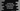# Javascript string indexOf() method explanation with example# Introduction :

Javascript indexOf() method is one of the most useful method of Javascript string. This method can be used to find out if a sub-string is included in a string or not. In this tutorial, we will learn how to use indexOf method with examples.

## Syntax :

The syntax of indexOf method is as below :

``````str.indexOf(subStr [, startIndex])
``````

### Parameters :

It takes two parameters, one is optional.

subStr : This is the sub string to find in the string str.

startIndex : This is an optional integer value to define the start index from where the search should begin. By default, it’s value is 0 i.e. the search starts from the first character of the string.

### Return value :

The return value is the index of the first occurrence of the substring found in the string. If the substring is not found, it will return -1. If the startIndex is passed, it will start the search from that index. The index of characters starts from 0 in Javascript string.

### Example :

Let’s take a look at the example below :

``````    let str = "The quick brown fox jumps over the lazy dog";

//1
console.log(str.indexOf("The"));

//2
console.log(str.indexOf("the"));

//3
console.log(str.indexOf("h"));

//4
console.log(str.indexOf("h",5));

//5
console.log(str.indexOf("hello"));
``````

### Output :

``````    0
31
1
32
-1
``````

### Explanation :

In this example :

1. The first console.log statement prints 0 because the first occurrence of ‘The’ is at index 0 in the string.
2. The second console.log statement prints 31 because the first occurrence of ‘the’ is at index 31 in the string.
3. The first occurrence of ‘h’ is at index 1.
4. The first occurrence of ‘h’ is at index 32 if the search starts at index 5 .
5. The substring ‘hello’ is not available in the given string. So, it prints -1 .

### Conclusion :

In this tutorial, we have learned how to use indexOf method in Javascript with examples. Try to run the examples shown above and drop one comment below if you have any queries.